Analyzing TwoVariable Data Lesson 2 1 Relationships Between

• Slides: 12Analyzing Two-Variable Data Lesson 2. 1 Relationships Between Two Categorical Variables Statistics and Probability with Applications, 3 rd Edition Starnes & Tabor Bedford Freeman Worth Publishers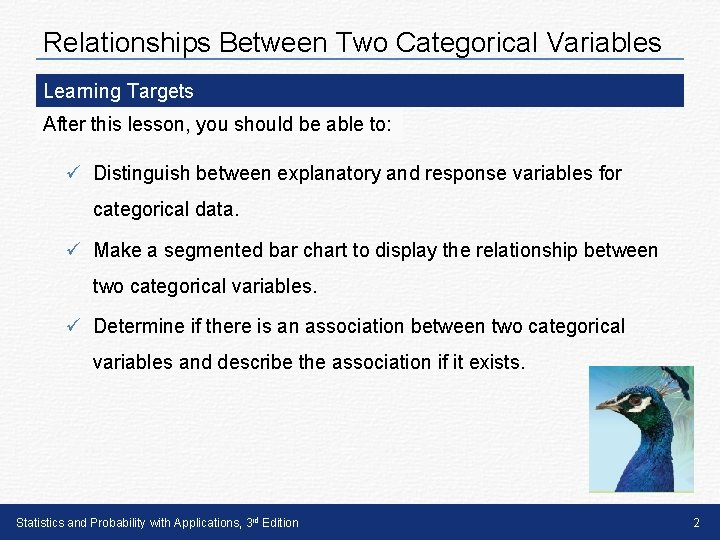Relationships Between Two Categorical Variables Learning Targets After this lesson, you should be able to: ü Distinguish between explanatory and response variables for categorical data. ü Make a segmented bar chart to display the relationship between two categorical variables. ü Determine if there is an association between two categorical variables and describe the association if it exists. Statistics and Probability with Applications, 3 rd Edition 2Relationships Between Two Categorical Variables The first step in describing relationships between two categorical variables is to determine which variable is the response variable and which variable is the explanatory variable. Response Variable, Explanatory Variable A response variable measures an outcome of a study. An explanatory variable may help predict or explain changes in a response variable. Note: In some relationships, there isn’t a clear explanatory or response variable. Statistics and Probability with Applications, 3 rd Edition 3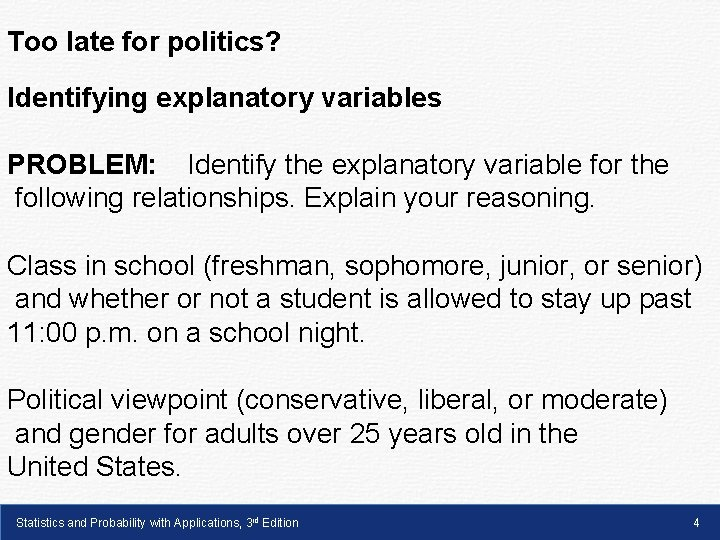Too late for politics? Identifying explanatory variables PROBLEM: Identify the explanatory variable for the following relationships. Explain your reasoning. Class in school (freshman, sophomore, junior, or senior) and whether or not a student is allowed to stay up past 11: 00 p. m. on a school night. Political viewpoint (conservative, liberal, or moderate) and gender for adults over 25 years old in the United States. Statistics and Probability with Applications, 3 rd Edition 4Relationships Between Two Categorical Variables After identifying the explanatory variable, the next step is to display the distribution of the response variable for each category of the explanatory variable. This can be done with side-by-side bar charts or with segmented bar charts. Segmented Bar Chart A segmented bar chart displays the possible values of a categorical variable as segments of a rectangle, with the area of each segment proportional to the percent of individuals in the corresponding category. Statistics and Probability with Applications, 3 rd Edition 5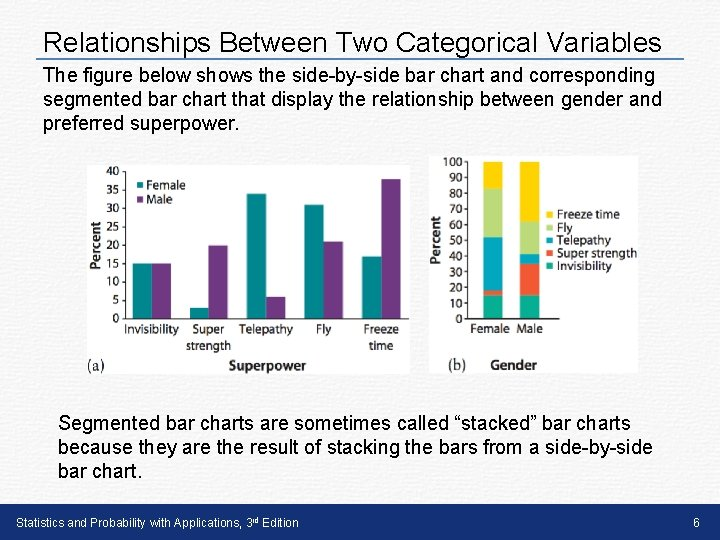Relationships Between Two Categorical Variables The figure below shows the side-by-side bar chart and corresponding segmented bar chart that display the relationship between gender and preferred superpower. Segmented bar charts are sometimes called “stacked” bar charts because they are the result of stacking the bars from a side-by-side bar chart. Statistics and Probability with Applications, 3 rd Edition 6Relationships Between Two Categorical Variables How to Make a Segmented Bar Chart 1. Identify the variables. Determine which variable is the explanatory variable and which is the response variable. 2. Draw and label the axes. Put the name of the explanatory variable under the horizontal axis. To the left of the vertical axis, indicate if the graph shows the percent (or proportion) of individuals in each category of the response variable. 3. “Scale” the axes. Write the names of the categories of the explanatory variable under the horizontal axis. On the vertical axis, start at 0% (or 0) and place tick marks at equal intervals until you reach 100% (or 1). 4. Draw “ 100%” bars above each of the category names for the explanatory variable on the horizontal axis. Make the bars equal in width and leave gaps between them. 5. “Segment” each of the bars. For each category of the explanatory variable, calculate the relative frequency for each category of the response variable. Then, divide the corresponding bar so that the area of each segment corresponds to the proportion of individuals in each category of the response variable. 6. Include a key that identifies the different categories of the response variable. Statistics and Probability with Applications, 3 rd Edition 7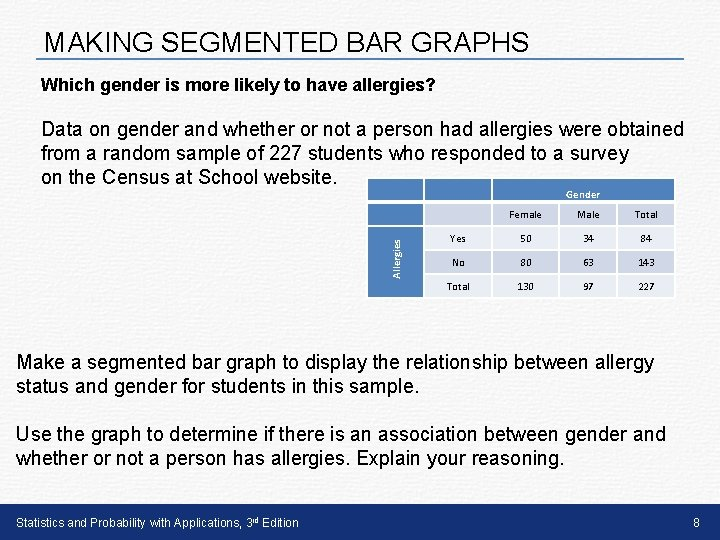MAKING SEGMENTED BAR GRAPHS Which gender is more likely to have allergies? Data on gender and whether or not a person had allergies were obtained from a random sample of 227 students who responded to a survey on the Census at School website. Allergies Gender Female Male Total Yes 50 34 84 No 80 63 143 Total 130 97 227 Make a segmented bar graph to display the relationship between allergy status and gender for students in this sample. Use the graph to determine if there is an association between gender and whether or not a person has allergies. Explain your reasoning. Statistics and Probability with Applications, 3 rd Edition 8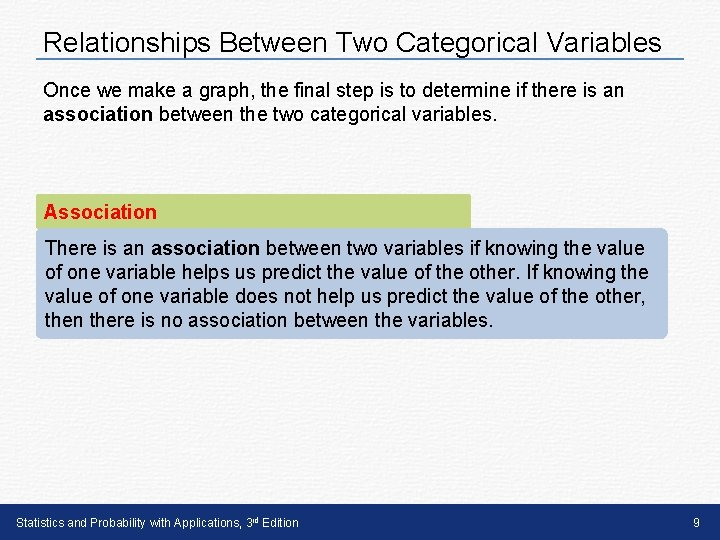Relationships Between Two Categorical Variables Once we make a graph, the final step is to determine if there is an association between the two categorical variables. Association There is an association between two variables if knowing the value of one variable helps us predict the value of the other. If knowing the value of one variable does not help us predict the value of the other, then there is no association between the variables. Statistics and Probability with Applications, 3 rd Edition 9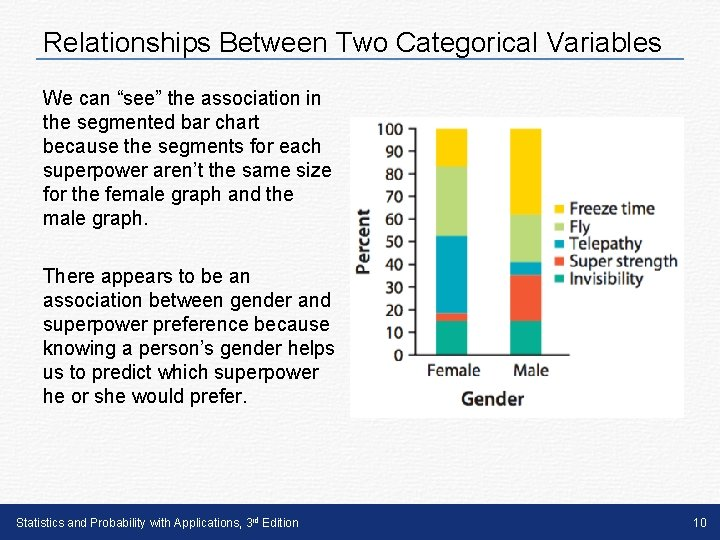Relationships Between Two Categorical Variables We can “see” the association in the segmented bar chart because the segments for each superpower aren’t the same size for the female graph and the male graph. There appears to be an association between gender and superpower preference because knowing a person’s gender helps us to predict which superpower he or she would prefer. Statistics and Probability with Applications, 3 rd Edition 10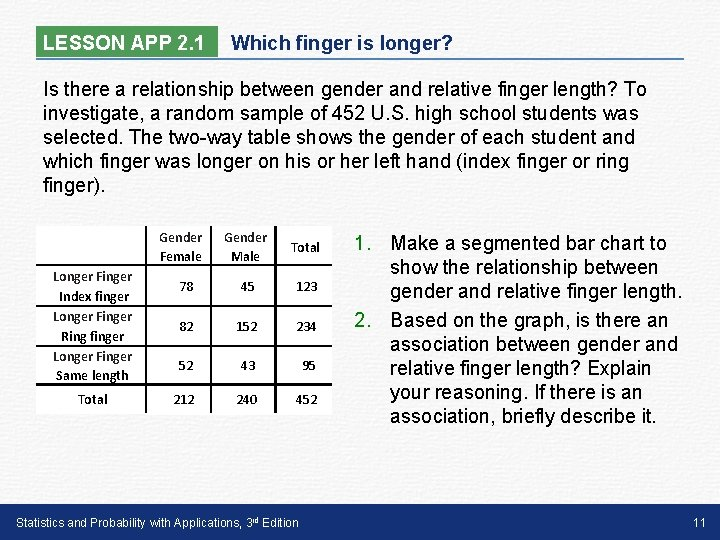LESSON APP 2. 1 Which finger is longer? Is there a relationship between gender and relative finger length? To investigate, a random sample of 452 U. S. high school students was selected. The two-way table shows the gender of each student and which finger was longer on his or her left hand (index finger or ring finger). Gender Female Longer Finger Index finger Longer Finger Ring finger Longer Finger Same length Total Gender Male Total 78 45 123 82 152 234 52 43 95 212 240 452 Statistics and Probability with Applications, 3 rd Edition 1. Make a segmented bar chart to show the relationship between gender and relative finger length. 2. Based on the graph, is there an association between gender and relative finger length? Explain your reasoning. If there is an association, briefly describe it. 11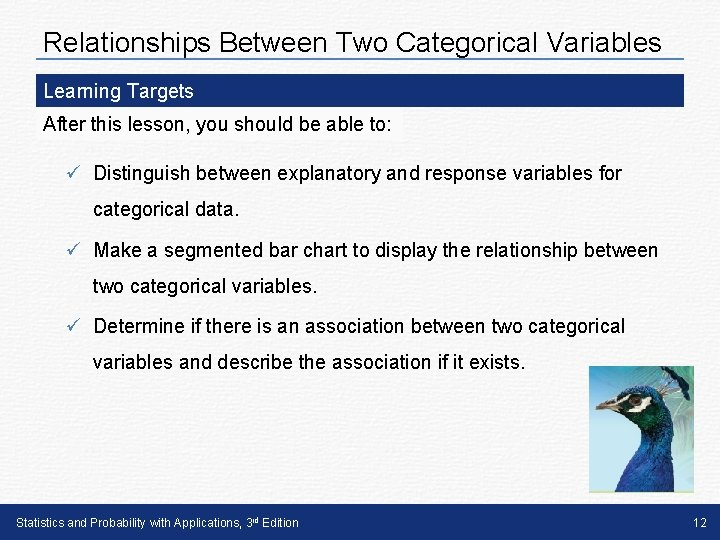Relationships Between Two Categorical Variables Learning Targets After this lesson, you should be able to: ü Distinguish between explanatory and response variables for categorical data. ü Make a segmented bar chart to display the relationship between two categorical variables. ü Determine if there is an association between two categorical variables and describe the association if it exists. Statistics and Probability with Applications, 3 rd Edition 12